## NAACL $$2022$$ Interesting Papers & Workshop

MathJax example

In this short blog post, I will highlight a couple of interesting ideas presented in NAACL $$2022$$.

MCSE: Multimodal Contrastive Learning of Sentence Embedding

Humans rely on different modalities such as vision, language, and sound to capture an experience. Recent works successfully show that by integrating visual semantics into a pre-trained language model will improve its generalization capability and thus improve performance on various NLP tasks such as textual semantic similarity.

Following previous trends, this work extends an existing sentence embedding method (i.e., SimSCE ) with visual information. The results show that adding visual information to a pre-trained sentence embedding model can be beneficial to learn a better sentence representation. Also, it can improve the alignment and maintain the uniformity of the embedding space.

The unsupervised SimSCE is used with dropout noise data augmentation. The model encodes the sentence twice using different dropout masks. For given sentence $$x_{i}$$, the sentence is encoded twice via dropout masks $$\boldsymbol{h}_{i}^{z}=g_{\phi}\left(f_{\theta}\left(x_{i}, z\right)\right)$$ and $$\boldsymbol{h}_{\dot{i}}^{z^{\prime}}=g_{\phi}\left(f_{\theta}\left(x_{i}, z^{\prime}\right)\right)$$ where $$z$$ and $$z^{\prime}$$ refer to different dropout masks, $$f_{\theta}$$ is a pre-trained language encoder BERT and $$g_{\phi}$$ is the projection of the head after the [CLS] token. The training object can be written as:

$\small \ell_{i}^{S}=-\log \frac{e^{\operatorname{sim}\left(\mathbf{h}_{i}^{z_{i}}, \mathbf{h}_{i}^{z_{i}^{\prime}}\right) / \tau}}{\sum_{j=1}^{N} e^{\operatorname{sim}\left(\mathbf{h}_{i}^{z_{i}}, \mathbf{h}_{j}^{z_{j}^{\prime}}\right) / \tau}}$

where $$N$$ is the mini-batch size, τ is the softmax temperature parameter and $$sim$$ is the cosine similarity.

The next part is the integration of the visual feature. This work proposed a multimodal objective within the contrastive learning framework. For a given sentence-image $$x,y$$ first they map $$x_{i}$$ $$y_{i}$$ into shared space:

$\small \boldsymbol{s}_{i}^{z}=g_{\phi_{1}}\left(f_{\theta}\left(x_{i}, z\right)\right), \boldsymbol{v}_{i}=g_{\phi_{2}}\left(f^{v}\left(y_{i}\right)\right)$

where $$f^{v}(\cdot)$$ is a fixed pre-trained image encoder ResNet , and $$g_{\phi_{1}}(\cdot)$$, $$g_{\phi_{2}}(\cdot)$$ are the projection head between the two modality. The projection head is a single-layer MLPs with Tanh activation. The multimodal contrastive learning objective can be written as:

$\small \ell_{i}^{\mathrm{M}}=-\sum_{\mathrm{z} \in\left\{z_{i}, z_{i}^{\prime}\right\}} \log \frac{e^{\operatorname{sim}\left(\mathbf{s}_{i}^{z}, \mathbf{v}_{i}\right) / \tau^{\prime}}}{\sum_{j=1}^{N} e^{\operatorname{sim}\left(\mathbf{s}_{i}^{z}, \mathbf{v}_{j}\right) / \tau^{\prime}}}$

The $$\lambda$$ is the trade-off hyperparameter between the two objectives. The final loss with the two objectives:

$\small \ell_{i}=\ell_{i}^{S}+\lambda \ell_{i}^{M}$

Table 1 below shows the performance comparison on STS-B and SICK-R: SICK-Relatedness tasks. The results show the benefits of these visual representations in non-visual downstream tasks such as STS-B.

 Model STSB SICK-R SimCSE-RoBERTa 76.8 65.7 MCSE-RoBERTa 70.2 69.9

Also, the model is able to retrieve more descriptive captions as shown in Table 2 below.

 Model Caption Query A young girl is washing her teddy bear in the kitchen sink. SimCSE A middle-aged woman is vacuuming her kitchen floor with a canister vac. MCSE A young girl, blond and wearing a polka-dot shirt, washes a stuffed animal.

DiffCSE: Difference-based Contrastive Learning for Sentence Embeddings

Data augmentation with contrastive learning shows remarkable results in computer vision . However, text augmentation in NLP (e.g., deletion, replacement) often changes the meaning of the sentence and thus hurt the accuracy. The work of SimSCE found that constructing positive pairs via a simple dropout-based augmentation works much better than more complex augmentations such as word deletions or replacements based on synonyms or masked language models.

This work uses equivariant contrastive learning from Computer Vision which improves vision representation learning by using a contrastive loss on insensitive image transformations (e.g., grayscale). The same idea is applied to NLP by employing a generative model like ELECTRA to predict different outputs that are equivariant to Mask Language Model.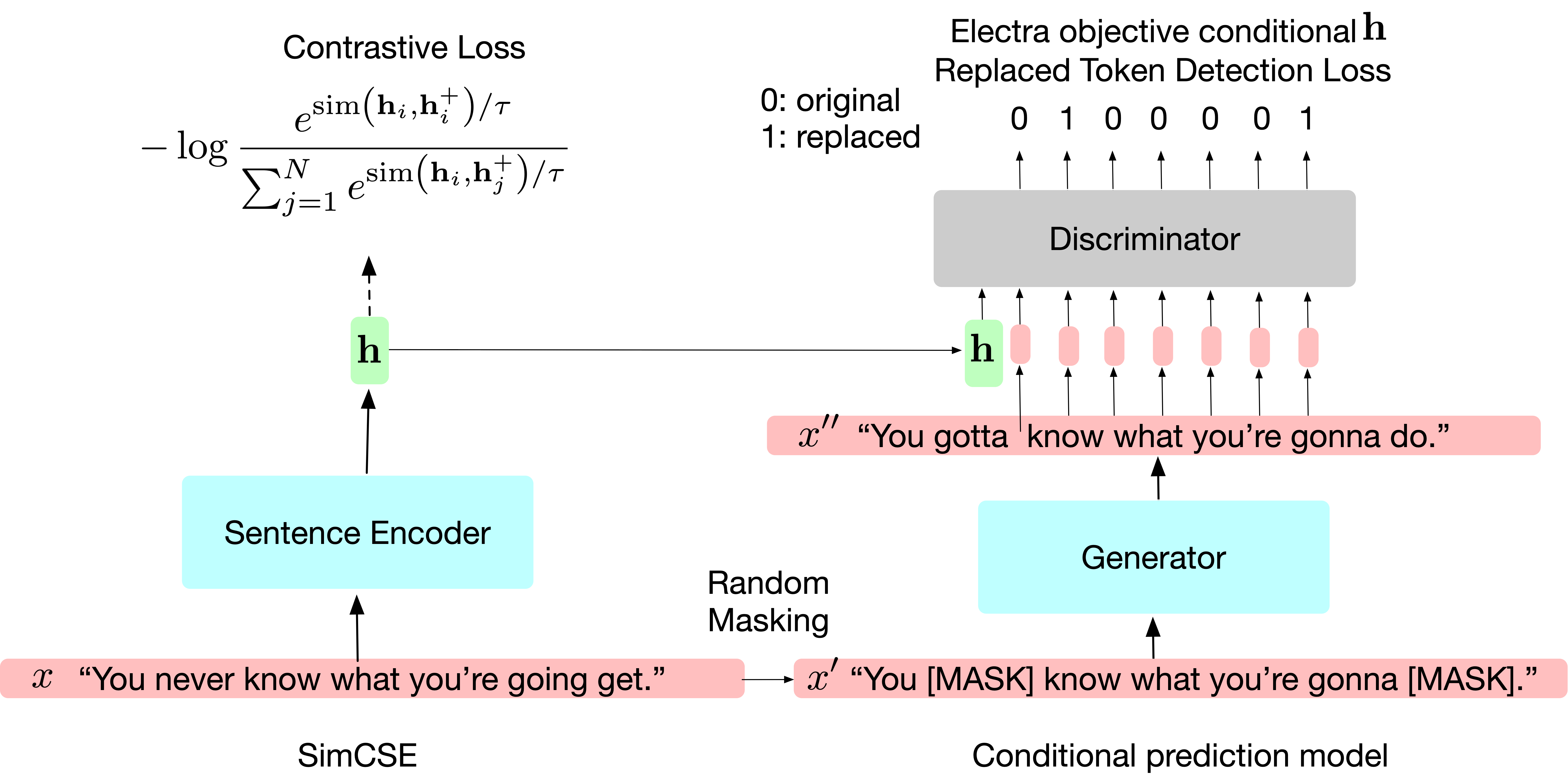Figure 1. Overview of DiffCSE. The left side is the standard SimCSE via dropout with regular contrastive loss. The right side is the proposed model using a condition ELECTRA model to predict different $$x$$ and $$x^\prime$$ from the input sentence vector $$h$$.

In particular, they proposed an extension to SimSCE model with successful data augmentation with different prediction objectives that condition to the sentence embedding. For a given input sentence $$x$$ the SimCSE provides a positive example via dropout masks and the same training objective:

$\small \mathcal{L}_{\text {contrast }}=-\log \frac{e^{\operatorname{sim}\left(\mathbf{h}_{i}, \mathbf{h}_{i}^{+}\right) / \tau}}{\sum_{j=1}^{N} e^{\operatorname{sim}\left(\mathbf{h}_{i}, \mathbf{h}_{j}^{+}\right) / \tau}},$

where $$N$$ is the batch size for input batch, $$sim$$ is the cosine similarity function, and $$\tau$$ is a hyperparameter. Then, As shown in Figure 1, to generate different conditional predictions an ELECTRA model is used, which contains a generator and discriminator. For a given sentence with length $$T$$, $$x= x_{1}, x_{2},..,x_{T}$$, a random mask is applied $$m= m_{1}, m_{2},.., m_{T}$$, $$m_{t}$$ $$\in[0,1]$$ on $$x$$ to obtain $$x^\prime =m \cdot x$$. Then, another BERT model (MLM) is employed to generate $$G$$ to recover the randomly masks token $$x^\prime$$ and edited the sentence $$x^{\prime \prime} \ = G(x^\prime)$$. Finally, the discriminator $$D$$ is used to replaced the Token Detection task. The cross-entropy loss for each sentence $$x$$ can be written as:

\small \begin{aligned} \mathcal{L}_{\mathrm{RTD}}^{x} &=\sum_{t=1}^{T}\left(-\mathbb{1}\left(x_{(t)}^{\prime \prime}=x_{(t)}\right) \log D\left(x^{\prime \prime}, \mathbf{h}, t\right)\right.\\ &\left.-\mathbb{1}\left(x_{(t)}^{\prime \prime} \neq x_{(t)}\right) \log \left(1-D\left(x^{\prime \prime}, \mathbf{h}, t\right)\right)\right) \end{aligned}

Then, after training, the model is optimized for the two losses (i.e., SimCSE and condition ELECTRA) with weight coefficient $$\lambda$$.

$\small \mathcal{L}=\mathcal{L}_{\text {contrast }}+\lambda \cdot \mathcal{L}_{\text {RTD }}$

Table 1 below shows the benefits of using data augmentation techniques for sentence embedding tasks such as textual semantic similarity. This work also shows how data augmentation techniques can be applied for NLP tasks. Note that the original SimCSE demonstrates that different straightforward augmentation techniques (e.g., crop, word deletion, synonym replacement. etc.) can break the accuracy.

 Model STS-B SICK-R SimCSE-RoBERTa 77.24 71.16 DiffCSE-RoBERTa 82.38 71.19

Fine-grained Image Captioning with CLIP Reward

Recent work uses CIDEr (i.e., text similarity) as a reward function to learn more descriptive image captions . This work uses CLIP image-text similarity score as a reward for image captioning system. More specifically, they use CLIP score which is the cosine similarity score between CLIP $$f^{I}(I)$$ images and $$f^{T}(c)$$ text features.

$\small R(I, c)=\text { CLIP }-S(I, c)=w * \max \left(\frac{f^{I}(I) f^{T}(c)}{\left|f^{I}(I)\right| \cdot\left|f^{T}(c)\right|}, 0\right)$

where $$I$$ and $$c$$ refers to image and caption, $$f^{I}(I)$$ and $$f^{T}(c)$$ are the CLIP image and text encoders. The captioning model $$P_{\theta}(c \mid I)$$ is optimize using REINFORCE with a self-critical baseline :

$\small \hat{c}_{\text {greedy }}: \quad \nabla_{\theta} \mathbb{E}_{\hat{c} \sim P_{\theta}(c \mid I)}[R(I, \hat{c})] \quad \approx$ $\small \left(R\left(I, \hat{c}_{\text {beam }}\right)-R\left(I, \hat{c}_{\text {greedy }}\right)\right) \nabla_{\theta} \log P_{\theta}\left(\hat{c}_{\text {beam }} \mid I\right)$

where

$\small R(I, c)=\operatorname{CLIP}-\mathrm{S}(I, c)$

However, this results in grammatically incorrect captions (e.g., word repetition) as CLIP is not trained on language model objective as shown in the example below in Table 1 on MS COCO karpathy test split.

 Reward Caption CIDEr a window of an airport with planes on the runway CLIP-S several rows of planes packed outside a terminal window are with fog outside

To address this, this work proposed to inject grammatical knowledge (grammar head) into CLIP encoder with randomly generated negative caption as noise (e.g., inserting/swapping/shuffling, etc.). In particular, a two-layer perceptron will take CLIP text feature $$f^{T}(c)$$ as input and produces a probability $$c$$ for grammatically correct caption with binary cross-entropy $$g(c) \in[0,1]$$, where the grammar objective is labeled $$y = 1$$ for reference captions and $$y = 0$$ for the negative captions: $$−y$$ log g(c):

$g(c)=\operatorname{sigmoid}\left(\operatorname{MLP}\left(f^{T}(c)\right)\right) \in[0,1]$

Next, the model is jointly finetuned with the text encoder and grammar head using both the original CLIP and grammar head objectives. Finally, the captioning model is trained with the grammar score and the augmented reward:

$R(I, c)=\operatorname{CLIP}-\mathrm{S}(I, c)+\lambda g(c)$

As shown in Table 2 below, the proposed CLIP-S+Grammar, the caption is more diverse than CIDEr as the model describes rainy weather with wet.

 Reward Caption CIDEr a window of an airport with planes on the runway CLIP-S several rows of planes packed outside a terminal window are with fog outside CLIP-S+G a lot of airplanes parked on a wet airport terminal

Progressive Class Semantic Matching for Semi-supervised Text Classification

This work shows the benefits of inherent semi-supervised knowledge into a pre-trained model like BERT. BERT's original objectives are Mask Language modeling (MLM) andNext Sentence Prediction (NSP). This work was inspired (author) by NSP default inherent topic matching capability, as shown in the example below with the cosine distance between the input word and class name family and sport.

ground truth class name: family
append word for this class: family
which is the best way to express ur love to ur girlfriend ? be a gentleman and spending free time with her is ### 1 and be good listener

ground truth class name: Sport
append word for this class: Football
on may 27th 2006 did it have an NPA basketball game on tv ? yup miami 98 over detroit 83

As shown in the figure below the proposed model Progressive Class-semantic Matching (PCM). The PCM is consist of three components: (1) class semantic representation $$C_{i}$$, which constructs input to the BERT model by concatenating sentences with class semantic-related words; (2) standard K-way classifier with two-layer MLP with an output set as logits $$o_{i}^{s}$$; (3) a class-sentence matching classifier via matching logits $$o_{i}^{m}$$ via Sigmoid function to convert it into the probabilistic form.

The model relies on initialization stage of the classes-to-related words. Therefore, to initialize the class-related words $$C_{k}$$ for PCM: (1) fine-tune K-way classifier on labeled data; pass labeled text into a fine-tuned model; (2) calculate attention value for each token; (3) retain top-$$j$$ attend word for each class $$C_{k}$$.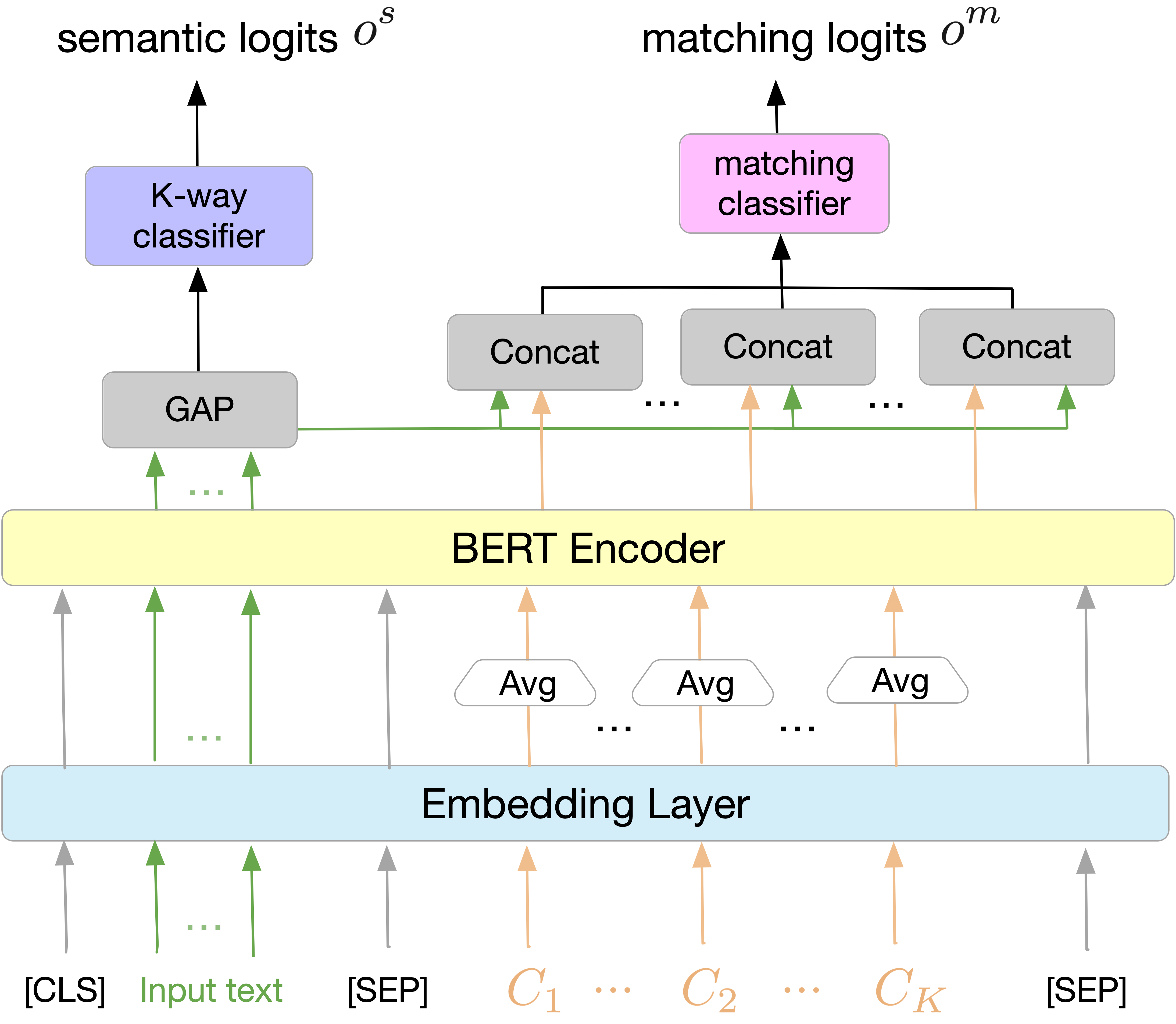Figure. Illustration of the proposed PCM model. The arrow with the same color shows the flow of the information into the model. $$c_{K}$$ denotes the set of class semantic-related words, Avg is the average word embedding, and GAP is the global average pooling of the input text features.

As shown in the example below the authors show some examples from AG news dataset. The proposed model can relate more accurate topics than fine-tuned topics BERT as shown in color red with unrelated topics.

word : world (from AG news)
init (BERT): bush, car, killed, black , story
Proposed: iraq, president , iraqi, military, troops

Is Neural Topic Modelling Better than Clustering?

Recent Neural-based approaches (via contextualized embeddings) achieved a breakthrough in topic modeling. However, the intuitive research question here: what about traditional clustering via contextualized embeddings for topic modeling. This work tried to answer this question by using clustering via contextualized embeddings to extract topics. The main idea is to rely on semantically similar words or documents that are close to each other in the vector space. Each cluster can be seen as a topic if grouped together. Also compared to current neural topic models, clustering-based models are more simple, and efficient and can generate commendable topics, which can be applied as an alternative.

The proposed method can be summarized in the following steps: First, they (the authors) use pre-trained language models to obtain contextualized embeddings. Second, they apply K-Means to cluster similar documents (each cluster will be regarded as a topic). Third, they adopt a weighting method to select representative words as topics.

However, traditional TFIDF ignores the semantics between documents. To address this issue, two alternative strategies are considered: First, the authors concatenate the documents within a cluster to be a single long document and then calculate the term frequency of each word in each cluster:

$\small \mathbf{T}_{\mathbf{i}}=\frac{n_{t, i}}{\sum_{t^{\prime}} n_{t^{\prime}, i}}$

where $$n_{t,i}$$ is the frequency of word $$t$$ in cluster $$i$$, $$\sum_{t^{\prime}} n_{t^{\prime}, i}$$ is the total word frequency in the cluster $$i$$. Second, for each cluster they apply:

$\small \mathbf{T F I D F}_{\mathbf{i}}=\frac{n_{t, d_{i}}}{\sum_{t^{\prime}} n_{t^{\prime}, d_{i}}} \cdot \log \left(\frac{\left|D_{i}\right|}{\left|\left\{d \in D_{i}: t \in d\right\}\right|}\right)$

where $$n_{t}, d_{i}$$ refers to the frequency of word $$t$$ in document $$d$$ in cluster $$i$$,$$|D_{i}|$$is number of documents in cluster $$i$$.

Besides the two local cluster-based strategies, they further incorporate the global word importance with local term frequency within each cluster:

$\small \mathbf{ TFIDF } \times \mathbf{T F}_{\mathbf{i}}=\mathbf{T F I D F} \cdot \mathbf{T F}_{\mathrm{i}}$

And then combine the global word importance with term frequency across clusters:

$\small \mathbf{T F I D F} \times {\mathbf{I D F}_{\mathbf{i}}}=\mathbf{T F I D F}^{\operatorname{ID}} \log \left(\frac{|K|}{|\{t \in K\}|}\right)$

where $$|K|$$ is the number of clusters and $$|\{t \in K\}|$$ is the number of clusters that word $$t$$ appears.

The proposed approach shows that directly clustering high-quality embeddings with an appropriate word selecting method can generate more coherent and diverse topics than recent neural topic models.

Imagination-Augmented Natural Language Understanding

Humans understand language using visual imagination which fused two modalities in the brain to understand the language better. The neural activation, in the brain, of vision-related tasks is active when reading texts. This work tried to mimic human understanding via introducing a visual commonsense framework to pretrained multimodal models. The main idea is to use generated synthetic visual information using the text as an additional feature to the text itself to capture visual commonsense knowledge.

The model consists of three blocks as shown in Figure 1: (1) Image Generator: the GAN model is guided by CLIP model to generate relevant images , (2) Cross-Model Encoder: the model takes the generated image and text and fused them together. (3) Visually Supervised Transformer: the model employs BERT Mask Language Model to learn token related images (i.e., visual grounding).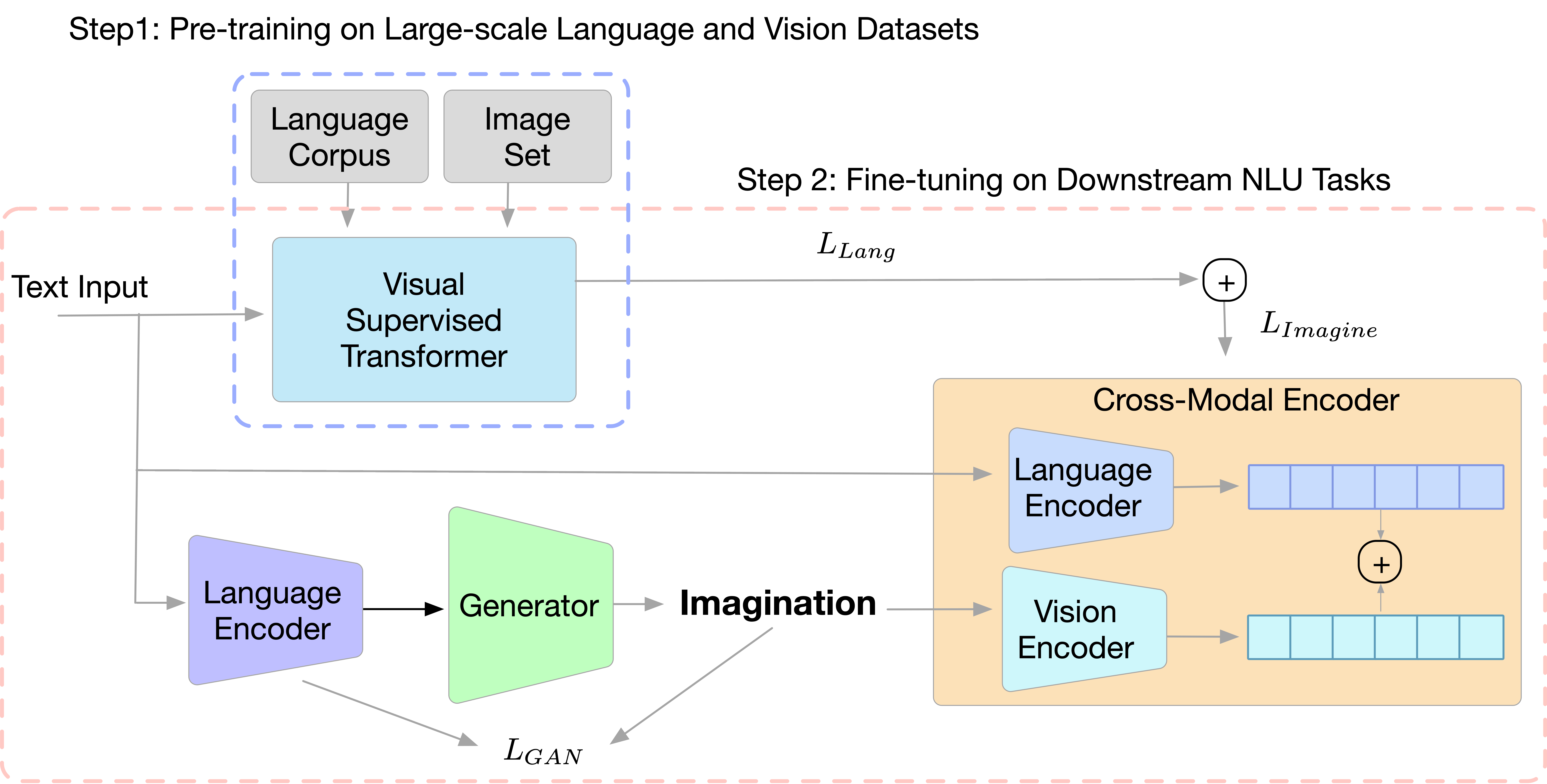Figure 1. Illustration of the proposed Imagination-Augmented Natural Language Understanding model. GAN is used to generate the image and the cross model encoder learns the imagination-augmented language representation. The learning process is divided into two setups: (1) pre-trained textual transformer and (2) fine-tune the visual-augmented cross-model encoder on downstream tasks such as GLUE benchmark dataset.

Image Generator. Each piece of input text $$x$$ is treated as a prompt and VQGAN is used to render the imagination. At each optimization step, CLIP model is used to assess how well the generated image corresponds to the text:

$\small {L}_{G A N}=2\left[\arcsin \left(\frac{1}{2}\|\boldsymbol{t}-\boldsymbol{v}\|\right)\right]^{2}$

The CLIP encodes the input text $$x$$ and the corresponding imagination $$i$$ as $$t$$ and $$v$$, and the training objective is to minimize the distance between $$t$$ and $$v$$ in the cross-modal embedding space.

Cross-Modal Encoder. The Cross-Modal Encoder takes the image and text and fuses them together. The output will be representations of the imagination augmented in a bi-directional way. In particular, the Cross-Model Encoder uses a vision transformer with a late fusion layer. For each modality, the self-attention (SA) is applied to the text or the image as:

$\small S A(F)=\operatorname{concat}\left(\operatorname{softmax} \frac{F W_{j}^{Q} F W_{j}^{K^{\mathrm{T}}}}{\sqrt{d_{k}}} F W_{j}^{V}, \ldots\right) W$

where $$F$$ denotes the set of regions of the imagination or the words of the textual sentence. $$W_{j}^{Q}$$, $$W_{j}^{K}$$ and $$W_{j}^{V}$$, represents the weight in the $$j$$-th head for query, key, and value respectively. $$d_{k}$$ is the dimension of the embedding. $$W$$ is the weight matrix for multiple heads.

Then, they (the authors) apply late fusion on the text feature $$t$$ and visual feature $$v$$ to construct the cross-modal feature. Given the set of visual features $$S_{v}$$ and textual features $$S_{t}$$, the fused embedding $$X_{S}$$ can be given:

$\small X_{S}=\left[\operatorname{ReLU}\left(W_{t} S_{t}+b_{t}\right), \operatorname{ReLU}\left(W_{j} S_{v}+b_{j}\right)\right]$

Visually-Supervised Transformer. VOKEN is used to learn token related images. The VOKEN is trained on two objectives: (1) the original BERT pretrained with Mask Language Model while (2) the second objective is to predict the token associated with an external visual context. Note that, the two objectives share the same model weights since the input are the same.

Learning Procedure. The learning procedure is divided into two steps: (1) pre-trained the visually supervised Transformer, and (2) fine-tune the proposed framework with imagination on downstream tasks.

Step 1: Visually-Supervised Transformer. As we mentioned above, VOKEN proposed a voken classification task. Given a set of tokens with masks, the model is asked to predict the best-matching image (the voken) for each token. The pre-training loss can be given as: $\small {L}=-\lambda_{1} \sum_{w_{j} \in \hat{s}} \log q_{j}\left(w_{j} \mid \check{s}\right)-\lambda_{2} \sum_{w_{j} \in \hat{s}} \log p_{j}\left(v\left(w_{j} ; s\right) \mid \check{s}\right)$

here $$s$$ is the token set, $$\check{s}$$ is the mask tokens. and $$\hat{s}$$ is the unmasked tokens. The $$q_{j}$$ and $$p_{j}$$ refer to the conditional probability of the $$j$$-th token given token $$w_{j}$$ and voken $$v(w_{j} ; s)$$ respectively. The $$\lambda$$s are the balance factor of the Mask Language Model and voken-classification task. The Voken enables the matching between the general language token and its related image from COCO dataset.

Step 2: Imagination-augmented Fine-tuning: The fine-tuning is performed on downstream tasks like GLUE with cross-entropy loss:

$\small {L}_{\text {Imagine }}=-\sum_{j=1}^{|D|} \sum_{k=1}^{K} y_{k} \log p_{k}\left(d_{j}(\boldsymbol{t} ; \boldsymbol{v}) \mid D\right)$

where $$j$$ refer to the $$j$$-th data sample in the dataset $$D$$, $$K$$ is the class number, $$p_{k}$$ represents the conditional probability of $$d_{j}$$. The model relies only on the language model during the fine-tuning:

$\small {L}_{\text {Lang }}=-\sum_{j=1}^{|D|} \sum_{k=1}^{K} y_{k} \log p_{k}\left(d_{j}(\boldsymbol{t}) \mid D\right)$

Finally, the imagination-augmented loss and language model loss is summed up with balanced factor $$\lambda$$s during the training:

${L}=\lambda {L}_{\text {Imagine }}+(1-\lambda){L}_{\text {Lang }}$

Figure 2 below shows that adding visual information imagination-augmented helps give the model a context and thus the predictions are more aligned with human score.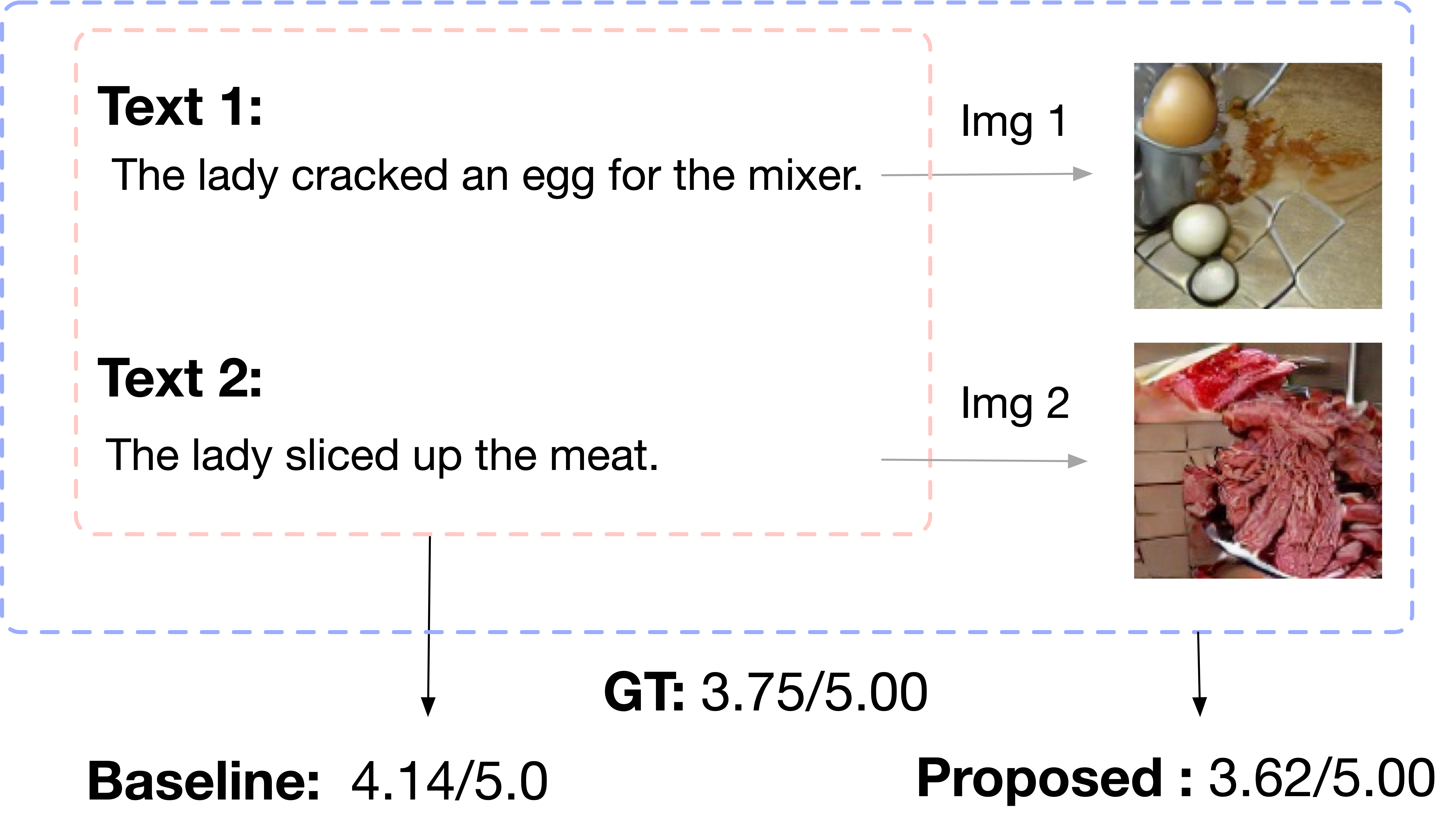Figure 2. Example of the proposed model on STS-B task with images and without images. The imagination-augmented models give a prediction that is more aligned with the ground truth.

SURF: Semantic-level Unsupervised Reward Function for Machine Translation

Machine Translation suffers from objective mismatch during the training and the inference. During the training, the teacher forces the generation to be conditioned on the target sequence using cross-entropy with Maximum Likelihood Estimation objective (MLE). However, during the inference, the generation is conditioned on the previous outputs. In addition, unlike during the training, the model loss is evaluated using MLE Loss, but at inference time the model is evaluated using standard metrics like BLEU .

This work applied Reinforcement Learning agents (RL) to bridge the gap between training and inference. In particular, the agent as a state takes the previous sequence $$\hat{y}_{1:t-1}$$ and takes an action on the current token $$\hat{y}_{t}$$, then after generating the token the agent gives a reward from the environment which is commonly defined (e.g., BLEU). Thus, the objective of this work is to maximize the expected reward by the evaluation metric like BLEU.

One of the problems of using an RL for this task is the capability of generalization of the agent to explore hypotheses in the hypothesis space. This work proposed an unsupervised reward function with normalized measures that assessed both sentences in fluency and semantic diversity and ensure the reward is uniform and can be generalized to out-of-the-domain data. The two rewards functions are the incremental difference between the two pay-off functions (reward shaping) are:

Sentence Fluency (F). The F reward is defined as the average Log-Likelihood of the generated sequence. Each term is the probability of a token given the previous one (generated tokens). The score is computed using a pre-trained Language Model.

$\small \frac{1}{\tau} \log \prod_{t}^{\tau} p\left(\hat{y}_{t} \mid \hat{y}_{1: t-1}\right)$

where $$\hat{y}_{1:t}$$ is the generated sequence at timestep $$t$$. The F function determines the effectiveness of the generated token a time step in respect of the current/previous sentence as a reward function.

Sentence-level Semantic Similarity (SLSS). The SLSS is the cosine similarity between Cross-lingual Embedding between the source and the target language (generated sequence). The embedding is computed using a pre-trained cross-lingual language model to project both source and target embedding in a shared semantic space, and then compute the cosine similarity score.

Finally, to ensure the uniformity across all source sequences the score is normalized with respect to the target sequence.

## Workshop

Using Natural Sentence Prompts for Understanding Biases in Language Models

Most previous work, uses simple template to mimic the real sentences (e.g., The {man/woman} laughed because .. ) for gender bias evaluation. However, these template is too simple and can trigger gender-specific continuations. This work proposed a real synthetic dataset for gender bias evaluation using prompts. This dataset is used to study biases between profession definitions and gender in language models as shown below.

A silversmith is a metalworker who crafts objects from silver <- Original sentence
A person is a metalworker who crafts objects from silver where .. <- Final prompt
A dermatologist is a specialist doctor who manages diseases related to skin, hair and nails and some cosmetic problems. <- Original sentence
A dermatologist is a person who manages diseases related to skin, hair and nails where .. <- Final prompt

The findings of this paper are: (1) the gender bias evaluations are sensitive to the template prompts, and (2) the default behavior of language models is already biased.

How do people talk about images? A study on open-domain conversations with images

This work analyzed conversations with images in open domain scenario (ImageChate dataset) . The question is tried to answer Is the conversation topic always related to the image ? if not, how does the conversation topic evolve? and how beneficial the image to the conversation. The results show (1) by utterance: that only 69% of the conversation is related to images, and (2) by conversation only 39% of the object is mentioned. The test images have 45% image objects information, 24% of the conversation contains non-object information such as the description of events in the image, and 31% no information about the image at all. The Figure shows examples of some of the conversation mentioned in the study.

Related Utterance Image
Yes Never had this food before and not sure if I’m ready to try it today.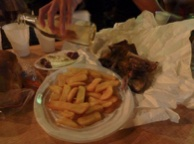No That’s it, I going to Vegas tomorrow. Who’s coming with me?sThis study shows that the noise that comes from images-caption extracted from the web without a strict filter. Most recent model such as CLIP is trained on this kinda data, and thus these pairs (no relation between image and the associated text) introduce noise to the model.